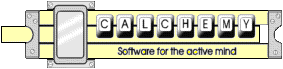## A Detailed Example

Suppose you want to calculate the required horsepower for a motor which could raise a 2000 lbf elevator to the top of a 10 story building (assuming 12 feet per story) in 1 minute, you could enter the equation:

```2000 lbf * (10 * 12 feet) / 1 minute ? horsepower
```
Indicating that you want to multiply 2000 pounds force by 10 times 12 feet, divide that by 1 minute, and then express the final result in horsepower. Calchemy would respond:
```= 7.27273 horsepower
```
If, on the other hand, you had made a mistake in the original equation and left out the "/ 1 minute":
```2000 lbf * (10 * 12 feet) ? horsepower
```
Calchemy would have responded with a Dimensional Mismatch dialog containing:
```Dimensional Mismatch [Frequency or Angular_velocity or Time^-1]
```
Indicating that the left hand side of the equation was missing a factor of 1/TIME -- the equation was not dimensionally correct, and therefore, could not be evaluated. Calchemy can also be used as a simple dimensionless calculator by eliminating the result unit from the equation. For example, you could enter the equation:
```2+3*5
```
Calchemy would respond with the algebraically correct answer:
```= 17
```
You could also enter the original equation in the following less explicit form:
```2000 lb; 10 stories; 12 feet/story; 1 minute ? horsepower
```
Indicating that you want to combine 2000 pounds, 10 stories, 12 feet/story, and 1 minute in such a way that the equation is dimensionally correct, and then express the final result in horsepower. Calchemy would again respond:
```= 7.27273 horsepower
```
This example illustrates the following features:

### Solving By Dimensional Analysis

The ";" operator tells Calchemy to use dimensional analysis to determine whether its operands should be in the numerator or the denominator of the equation. In this example, Calchemy placed the first three operands in the numerator and the last operand in the denominator. This allows you to be less involved with the actual mechanics of the equations.

The "lb" unit is an overloaded unit, meaning that it has more than one common definition. It is primarily defined as "lbm" (pounds mass) and secondarily defined as "lbf" (pounds force). Calchemy automatically uses the appropriate definition, again based on dimensional analysis. In this example, Calchemy used "lbf". This allows you to naturally use units with multiple common definitions.

### Free Units

The "stories" and "story" units are called free units. Free units are units which are not actually understood by Calchemy, except in that they are new and unique dimensions which must "cancel out" in the end. This allows you to enter equations in a more natural format.

### Pluralized Units

The "stories" and "story" units also illustrate that Calchemy understands basic pluralization rules for units. This, again, allows you to enter equations in a more natural format.

Calchemy Software Inc.
Fort Collins, COLast Updated 6/21/2009